Question

# Input voltage is applied to a circuit consisting of a series connection of capacitor C=10 uF...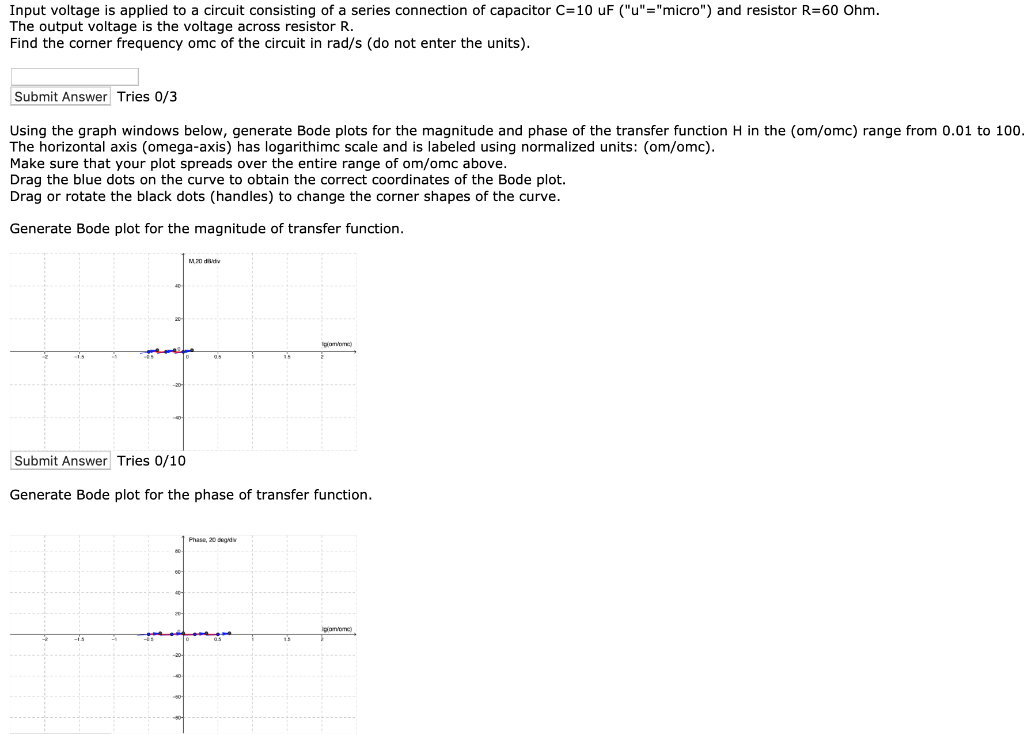Input voltage is applied to a circuit consisting of a series connection of capacitor C=10 uF ("u"="micro") and resistor R=60 Ohm. The output voltage is the voltage across resistor R. Find the corner frequency omc of the circuit in rad/s (do not enter the units). Submit Answer Tries 0/3 Using the graph windows below, generate Bode plots for the magnitude and phase of the transfer function H in the com/omc) range from 0.01 to 100- The horizontal axis (omega-axis) has logarithimc scale and is labeled using normalized units: (om/omc). Make sure that your plot spreads over the entire range of om/omc above. Drag the blue dots on the curve to obtain the correct coordinates of the Bode plot. Drag or rotate the black dots (handles) to change the corner shapes of the curve. Generate Bode plot for the magnitude of transfer function. Submit Answer Tries 0/10 Generate Bode plot for the phase of transfer function.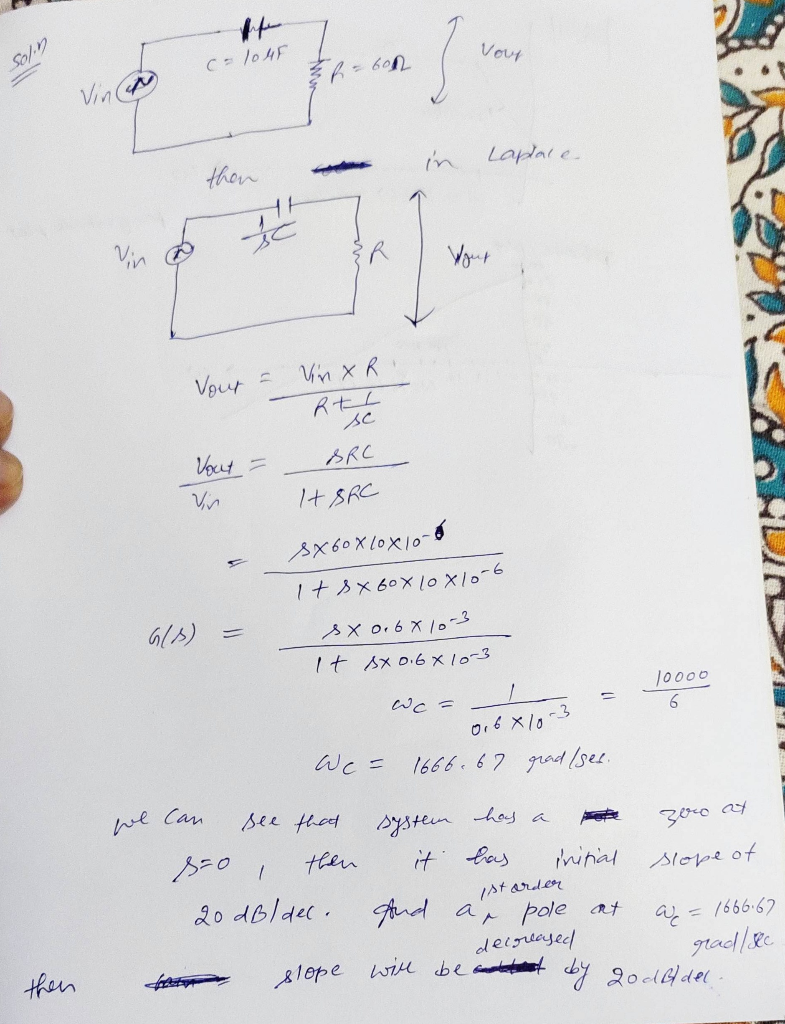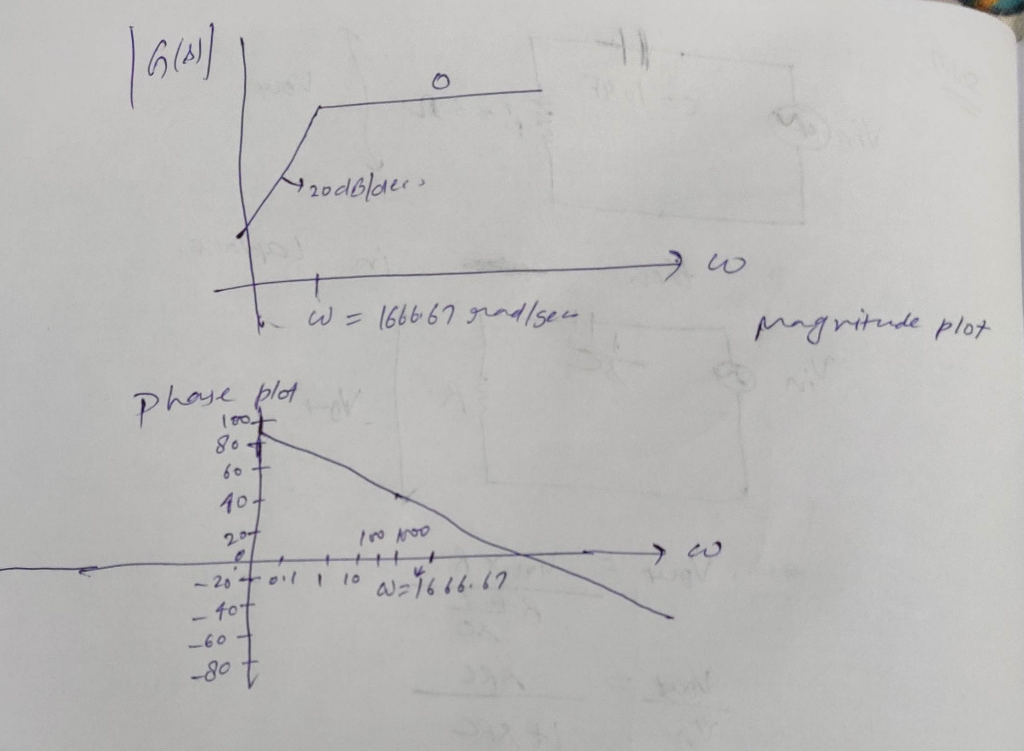#### Earn Coins

Coins can be redeemed for fabulous gifts.

Similar Homework Help Questions
• ### Consider the filter circuit (Figure 1) with R=500 N and C ==uF. Learning Goal: To understand...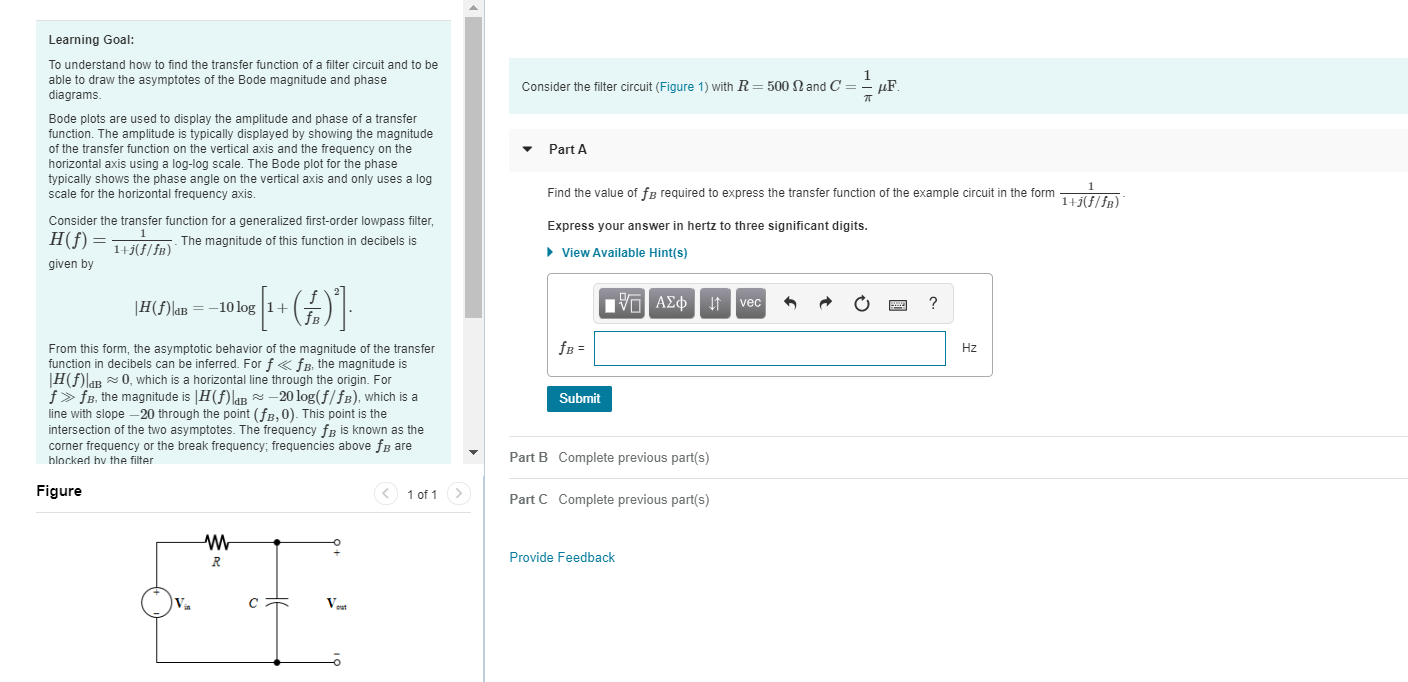Consider the filter circuit (Figure 1) with R=500 N and C ==uF. Learning Goal: To understand how to find the transfer function of a filter circuit and to be able to draw the asymptotes of the Bode magnitude and phase diagrams Bode plots are used to display the amplitude and phase of a transfer function. The amplitude is typically displayed by showing the magnitude of the transfer function on the vertical axis and the frequency on the horizontal axis using...

• ### 04. Given that R1-6000 ?, R,-22 ?, C1-4 uF, and C2-9.5 nF, for the Op-Amp circuit...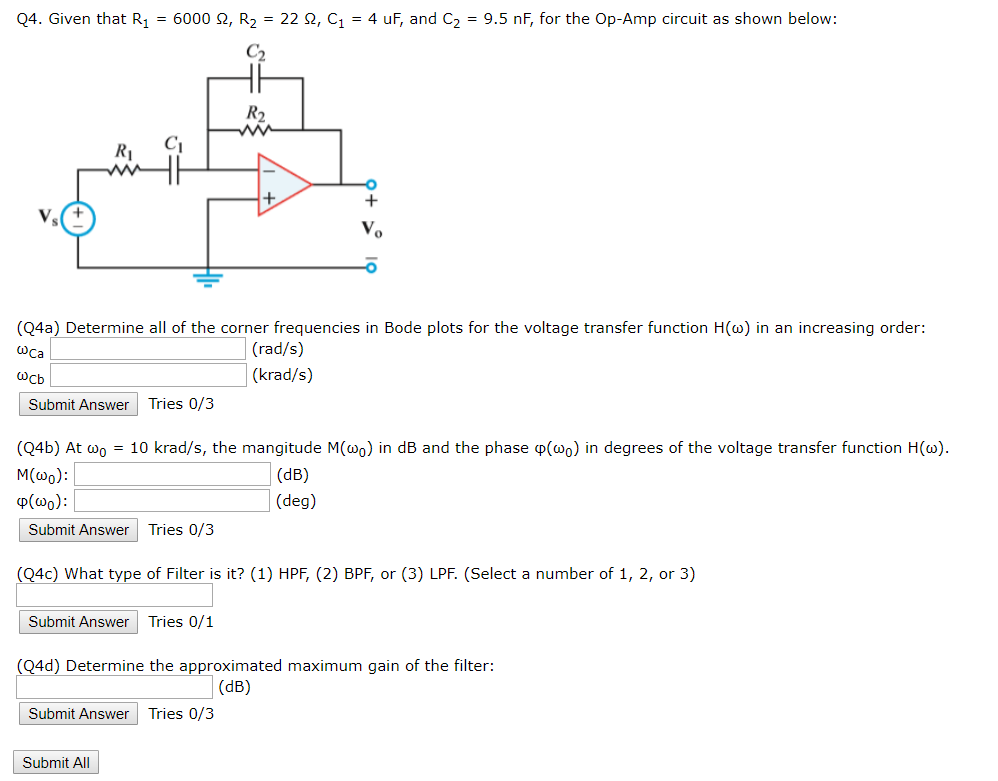04. Given that R1-6000 ?, R,-22 ?, C1-4 uF, and C2-9.5 nF, for the Op-Amp circuit as shown below: Ri (Q4a) Determine all of the corner frequencies in Bode plots for the voltage transfer function H() in an increasing order: Ca (rad/s) (krad/s) Submit Answer Tries 0/3 (04b) At ?0-10 krad/s, the mangitude M(wo) in dB and the phase ?(oo) in degrees of the voltage transfer function H(a) M(o): p(w0) (deg) Submit Answer Tries 0/3 Q4c) What type of Filter...

• ### A circuit has a 15022 resistor in series with a 10mH inductor. The voltage input is...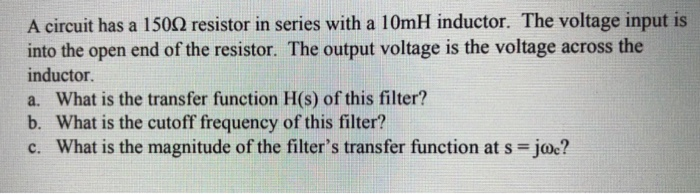A circuit has a 15022 resistor in series with a 10mH inductor. The voltage input is into the open end of the resistor. The output voltage is the voltage across the inductor a. What is the transfer function H(s) of this filter? b. What is the cutoff frequency of this filter? c. What is the magnitude of the filter's transfer function at s = joc?

• ### I need help with this  Bode Plots assignment (5 points) Follow the steps (a-c) described below for the following transf...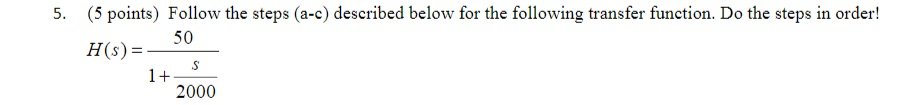I need help with this  Bode Plots assignment (5 points) Follow the steps (a-c) described below for the following transfer function. Do the steps in order! 5. H50 H(s) 2000 a. On a 'blank Bode plot' grid, plot the Bode plot straight line hand sketch approximation for magnitude for the frequency range from 1000 to ω.*1000. Be sure, though, that the corner frequency aligns with an appropriate vertical line on the grid. b. On graph paper, directly below the magnitude...

• ### I need help with this  Bode Plots assignment 2. (5 points) Follow the steps (a-c) described below for the following tra...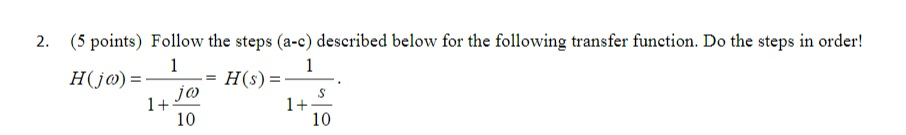I need help with this  Bode Plots assignment 2. (5 points) Follow the steps (a-c) described below for the following transfer function. Do the steps in order! 1+ 10 1+ 10 a. On a 'blank Bode plot' grid, plot the Bode plot straight line hand sketch approximation for magnitude for the frequency range from 1000 to ω.*1000. Be sure, though, that the corner frequency aligns with an appropriate vertical line on the grid. b. On graph paper, directly below the...

• ### 2. In the circuit below, R1 = 10k, R2 : magnitude is applied to the input....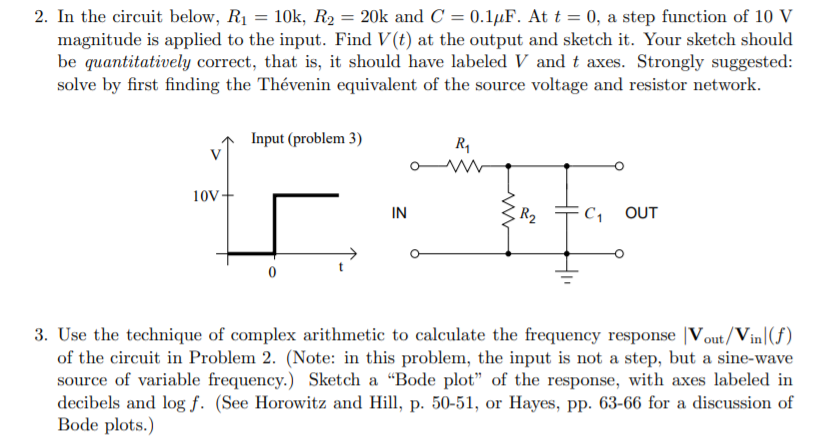2. In the circuit below, R1 = 10k, R2 : magnitude is applied to the input. Find V(t) at the output and sketch it. Your sketch should be quantitatively correct, that is, it should have labeled V and t axes. Strongly suggested: solve by first finding the Thévenin equivalent of the source voltage and resistor network. 20k and C = 0.1µF. At t = 0, a step function of 10 V Input (problem 3) R, 10V- IN R2 C, OUT...

• ### Consider the circuit below, where R-5000Ω,C-0.25μF . The voltage amplitude is 10 V and the frequency...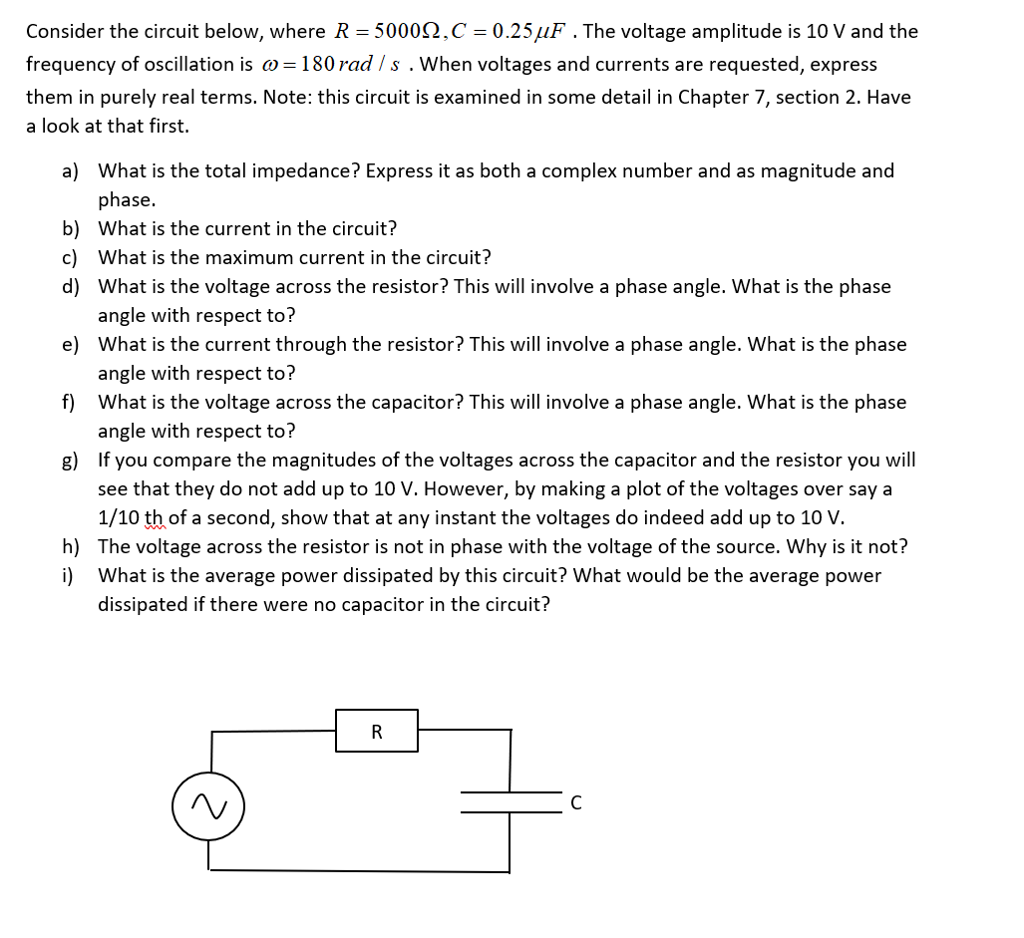Consider the circuit below, where R-5000Ω,C-0.25μF . The voltage amplitude is 10 V and the frequency of oscillation is 180 rad Is . When voltages and currents are requested, express them in purely real terms. Note: this circuit is examined in some detail in Chapter 7, section 2. Have a look at that first. a) What is the total impedance? Express it as both a complex number and as magnitude and phase. What is the current in the circuit? What...

• ### Please do question 524 the power in watts dissipated by the resistor. Hamad Alrobayan ans:4 ource, resistor, 524) Given a series circuit consisting of a DC voltage capacitor, inductor, and switch...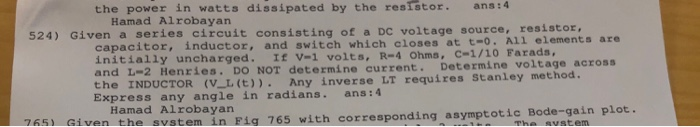Please do question 524 the power in watts dissipated by the resistor. Hamad Alrobayan ans:4 ource, resistor, 524) Given a series circuit consisting of a DC voltage capacitor, inductor, and switch which closes at t-o. All elements are initially uncharged. If v-1 volts, R-4 Ohms, C-1/10 Farads, and L-2 Henries. DO NOT determine current. Determine voltage across the INDUCTOR (VL(t)). Any inverse LT requires Stanley met Express any angle in radians. ans:4 Hamad Alrobayan 765 Given th vstem in Fig...

• ### 4. An electrical filter circuit is built with a series resistor (R), inductor (L) and capacitor...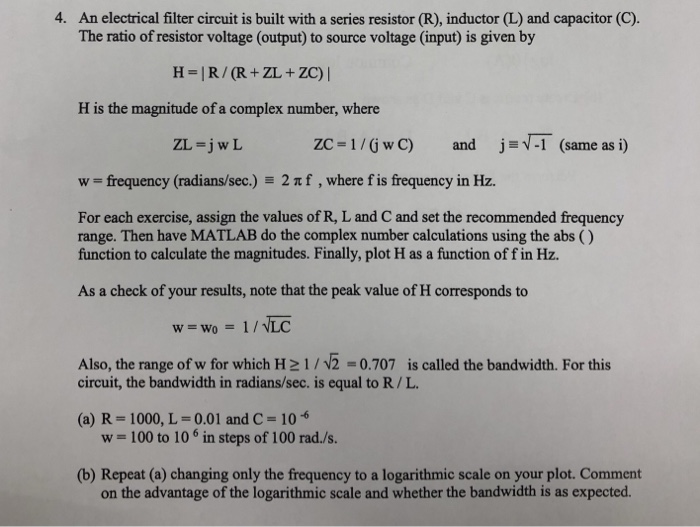4. An electrical filter circuit is built with a series resistor (R), inductor (L) and capacitor (C). The ratio of resistor voltage (output) to source voltage (input) is given by H is the magnitude of a complex number, where ZL=JwL ZC = 1 / (j w C) andjsVT (same as 1) w frequency (radians/sec) 2 f,where f is frequency in Hz. For each exercise, assign the values of R, L and C and set the recommended frequency range. Then have...

• ### Problem 05 In R Out For the circuit above, answer the following questions. 1. Derive the formula ...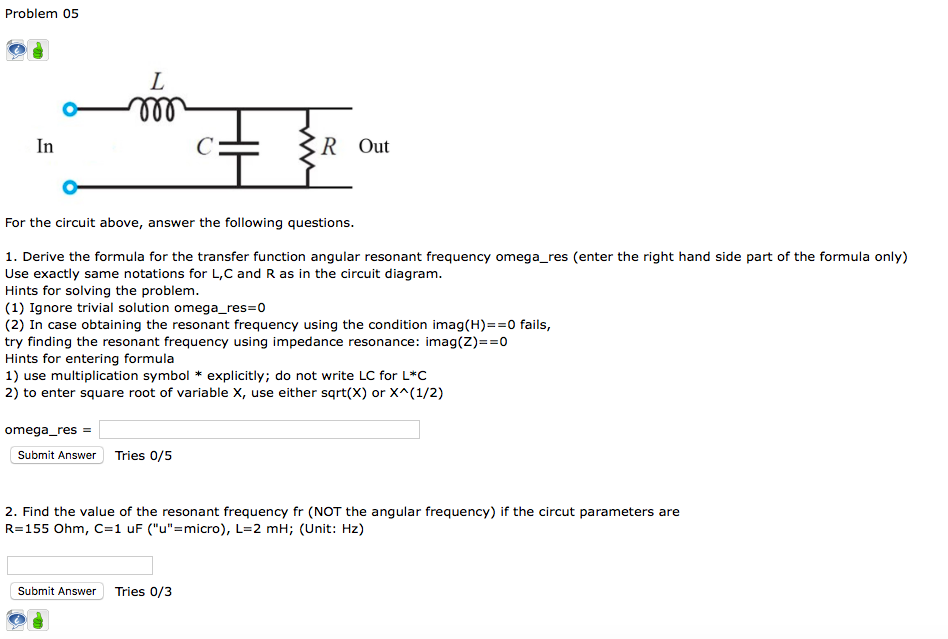Please Help! Problem 05 In R Out For the circuit above, answer the following questions. 1. Derive the formula for the transfer function angular resonant frequency omega_res (enter the right hand side part of the formula only) Use exactly same notations for L,C and R as in the circuit diagram. Hints for solving the problem. (1) Ignore trivial solution omega_res 0 (2) In case obtaining the resonant frequency using the condition imag(H)0 fails, try finding the resonant frequency using impedance...